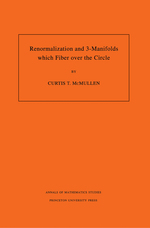# Renormalization and 3-Manifolds Which Fiber over the Circle (AM-142)

Curtis T. McMullen
Pages: 253
https://www.jstor.org/stable/j.ctt7ztfmd

1. Front Matter
(pp. i-iv)
(pp. v-x)
3. 1 Introduction
(pp. 1-10)

In the late 1970s Thurston constructed hyperbolic metrics on most 3-manifolds which fiber over the circle. Around the same time, Feigenbaum discovered universal properties of period doubling, and offered an explanation in terms of renormalization. Recently Sullivan established the convergence of renormalization for real quadratic mappings.

In this work we present a parallel approach to renormalization and to the geometrization of 3-manifolds which fiber over the circle. This analogy extends the dictionary between rational maps and Kleinian groups; some of the new entries are included in Table 1.1.

Both discussions revolve around the construction of a nonlinear dynamical system which...

4. 2 Rigidity of hyperbolic manifolds
(pp. 11-40)

This chapter begins with basic facts about complete hyperbolic manifolds and their geometric limits. We then give a proof of rigidity for manifolds whose injectivity radius is bounded above. Mostow rigidity for closed manifolds is a special case; the more general result will be used in the construction of hyperbolic manifolds which fiber over the circle.

The proof of rigidity combines geometric limits with the Lebesgue density theorem and the a.e. differentiability of quasiconformal mappings. This well-known argument is carried further in §2.4 to show certain open hyperbolic manifolds, while not rigid, areinflexible— any deformation is asymptotically isometric...

5. 3 Three-manifolds which fiber over the circle
(pp. 41-74)

This chapter presents the construction of hyperbolic 3-manifolds which fiber over the circle.

Consider a pseudo-Anosov homeomorphismψ:SSof a compact surface of negative Euler characteristic. To find a hyperbolic structure on the mapping torusTψofψ, one seeks a hyperbolic manifoldMψhomeomorphic to$S\times \mathbb{R}$on which the homotopy classψis represented by an isometryα. Then${{M}_{\psi }}/\left\langle \alpha \right\rangle$is homeomorphic toTψ.

It is easy to find hyperbolic structures on$S\times \mathbb{R}$such thatψis represented by a quasi-isometry. For example, a hyperbolic structure onSextends to$S\times \mathbb{R}$in a unique way...

6. 4 Quadratic maps and renormalization
(pp. 75-94)

We now turn from Kleinian groups and mapping classes to quadratic maps and renormalization.

Letf(z) =z2+cbe a quadratic polynomial. The most interesting behavior offis associated with the orbit of its critical pointz= 0. Suppose an iteratefnmaps a diskUncontaining 0 over itself properly by degree two, with imageVn. Thenfn:UnVnis aquadratic-like map, of the same general form asfitself. If the critical point remains inUnunder iteration offn, thenfn:UnVnis arenormalizationoffn. The...

7. 5 Towers
(pp. 95-104)

We now broaden our scope and considertowersof quadratic-like maps compatible under renormalization. These towers arise naturally as geometric limits of infinitely renormalizable quadratic polynomials. (This statement is made precise in §9.4.)

Alevel set$S\subset {{\mathbb{Q}}^{+}}$is a collection of positive rational numbers containing 1, such that for any pairs<tinS,t/sis an integer.

Atower$\mathcal{T}$is a collection of quadratic-like maps$\left\langle {{f}_{s}}:{{U}_{s}}\to {{V}_{s}};s\in S \right\rangle$such that:

1.Sis a level set;

2. the critical point of eachfs(z) is atz= 0;

3. the filled JuliaK(fs) is connected; and

4. for any levelss,twithn...

8. 6 Rigidity of towers
(pp. 105-118)

Let$\mathcal{T}=\left\langle {{f}_{s}}:{{U}_{s}}\to {{V}_{s}};s\in S \right\rangle$and${{\mathcal{T}}^{\prime }}=\left\langle {{g}_{s}}:U_{s}^{\prime }\to V_{s}^{\prime };s\in S \right\rangle$be a pair of towers with the same level setS. Aconjugacy ϕbetween$\mathcal{T}$and${{\mathcal{T}}^{\prime }}$is a bijection$\phi :\bigcup{{{V}_{s}}}\to \bigcup{V_{s}^{\prime }}$such that$\phi \circ {{f}_{s}}={{g}_{s}}\circ \phi$for all$s\in S$. A conjugacy may be conformal, quasiconformal, smooth, etc. according to the quality ofϕ.

A tower$\mathcal{T}$isquasiconformally rigidif any quasiconformal conjugacyϕfrom$\mathcal{T}$to another tower${{\mathcal{T}}^{\prime }}$is conformal.

Here are two equivalent formulations of quasiconformal rigidity which make no reference to${{\mathcal{T}}^{\prime }}$. AnLBeltrami differential$\mu =\mu (z)d\bar{z}/dz$on$\bigcup {{V}_{s}}$is$\mathcal{T}$-invariantif$f_{s}^{*}(\mu )=\mu |{{U}_{s}}$for all$s\in S$. Then we...

9. 7 Fixed points of renormalization
(pp. 119-134)

In this chapter we study the convergence of iterated renormalization over the complex numbers.

We begin by defining the renormalization operators${{\mathcal{R}}_{p}}$and discussing their relation to tuning and the Mandelbrot set. Then we give a conjectural construction of fixed points of renormalization, starting from purely combinatorial data, namely a quadratic polynomial with a periodic critical point. The construction parallels the geometrization of 3-manifolds that fiber over the circle.

Next we justify several steps in the construction, starting with a quadratic polynomialfwhose inner class is fixed under renormalization. We show that if the quadratic germs of$\mathcal{R}_{p}^{n}(f)$have...

10. 8 Asymptotic structure in the Julia set
(pp. 135-150)

Letf(z) =z2+cbe an infinitely renormalizable quadratic polynomial with bounded combinatorics and definite moduli. That is, suppose there are constantsBandm> 0 such that$\mathcal{S}\mathcal{R}(f)=\{{{n}_{0}},{{n}_{1}},{{n}_{2,}}\ldots \}$satisfies supni+1/niBfor alli, and mod(f,n) ≥m> 0 for all$n\in \mathcal{S}\mathcal{R}(f)$.

By Sullivan’sa prioribounds, anyrealinfinitely renormalizable quadratic polynomial with bounded combinatorics automatically has definite moduli. In particular, the Feigenbaum polynomial is an example of such anf.

In this section we collect together some additional results about the geometry and dynamics of these polynomials. We show the complement...

11. 9 Geometric limits in dynamics
(pp. 151-174)

In this chapter we formulate a general theory of holomorphic dynamical systems. The setting will be broad enough to include both Kleinian groups and iterated rational maps. Its scope will also encompass geometric limits of rational maps; as we have seen, renormalization naturally leads from polynomials to polynomial-like maps, and it is useful to allow quite general transformations in the limiting dynamics.

We then prove inflexibility theorems for dynamical systems which generalize our earlier results for Kleinian groups. The injectivity bounds in Theorems 2.11 and 2.18 are replaced by the assumption ofuniform twisting, formulated in §9.3 below.

As an...

12. 10 Conclusion
(pp. 175-182)

In this chapter we briefly recapitulate the analogies between the action of a mapping classψ:AH(S) →AH(S) and the action of a renormalization operator${{\mathcal{R}}_{p}}:{{\mathcal{H}}^{(p)}}\to \mathcal{H}$. We also discuss some open problems on both sides of the dictionary. These parallels and problems were summarized in Tables 1.1 and 1.2 of §1.

Consider first the algorithms for the construction of fixed points, detailed in §3.4 forψand in §7.1 for${{\mathcal{R}}_{p}}$. In each case, the dynamics at the fixed point seems to be hyperbolic, with both expanding and contracting directions. The fixed point cannot be located...

13. Appendix A. Quasiconformal maps and flows
(pp. 183-204)
14. Appendix B. Visual extension
(pp. 205-240)
15. Bibliography
(pp. 241-250)
16. Index
(pp. 251-253)